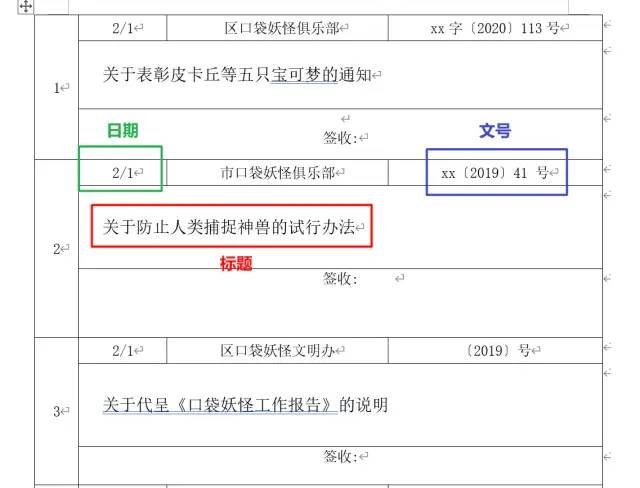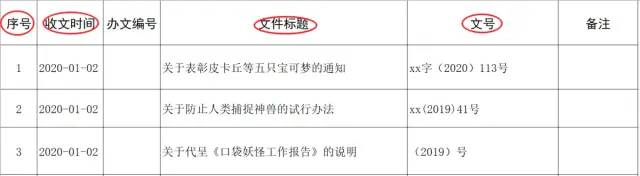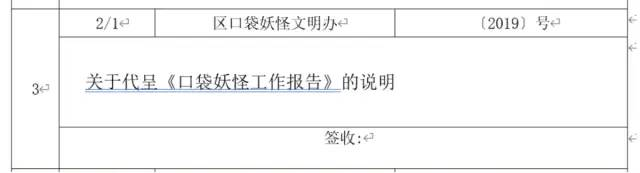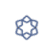# aspose word 获取标题_如何利用Python批量将Word中的信息提取到Excel？• 日期

• 发文单位

• 文号

• 标题

• 签收栏# 导入需要的库docxfrom docx import Document# 指定文件存放的路径path = r'C:\Users\word.docx' # 读取文件document = Document(path)# 读取word中的所有表格tables = document.tables

# 获取第一张表table0 = tables# 在全局放一个变量用来计数填序号n = 0for i in range(0, len(table0.rows) + 1, 3):    # 日期    date = table0.cell(i, 1).text    # 标题    title = table0.cell(i + 1, 1).text.strip()    # 文号    dfn = tables[j].cell(i, 3).text.strip()    print(n, date, tite, dfn)

• strptime: 解析字符串中蕴含的时间

• strftime: 转化成所需的时间格式

import datetimen = 0for i in range(0, len(table0.rows) + 1, 3):    # 日期    date = table0.cell(i, 1).text    # 有的条目时间是空的，这里不做过多判别    if '/' in date:        date = datetime.datetime.strptime(date, '%d/%m').strftime('2020-%m-%d')    else:        date = '-'    # 标题    title = table0.cell(i + 1, 1).text.strip()    # 文号    dfn = tables[j].cell(i, 3).text.strip()    print(n, date, tite, dfn)

n = 0for j in range(len(tables)):    for i in range(0, len(tables[j].rows)+1, 3):        try:            # 日期            date = tables[j].cell(i, 1).text            if '/' in date:                date = datetime.datetime.strptime(date, '%d/%m').strftime('2020-%m-%d')            else:                date = '-'            # 标题            title = tables[j].cell(i + 1, 1).text.strip()            # 文号            dfn = tables[j].cell(i, 3).text.strip()            n += 1            print(n, date, title, dfn)        except Exception as error:            # 捕获异常，也可以用log写到日志里方便查看和管理            print(error)            continue

from openpyxl import Workbook# 实例化wb = Workbook()# 获取当前sheetsheet = wb.active# 设立表头header = ['序号', '收文时间', '办文编号', '文件标题', '文号', '备注']sheet.append(header)

row = [n, date, ' ', title, dfn, ' ']sheet.append(row)

wb.save(r'C:\Users\20200420.xlsx')

from docx import Documentimport datetimefrom openpyxl import Workbookwb = Workbook()sheet = wb.activeheader = ['序号', '收文时间', '办文编号', '文件标题', '文号', '备注']sheet.append(header)path = r'C:\Users\word.docx'document = Document(path)tables = document.tablesn = 0for j in range(len(tables)):    for i in range(0, len(tables[j].rows)+1, 3):        try:            # 日期            date = tables[j].cell(i, 1).text            if '/' in date:                date = datetime.datetime.strptime(date, '%d/%m').strftime('2020-%m-%d')            else:                date = '-'            # 标题            title = tables[j].cell(i + 1, 1).text.strip()            # 文号            dfn = tables[j].cell(i, 3).text.strip()            n += 1            print(n, date, title, dfn)            row = [n, date, ' ', title, dfn, ' ']            sheet.append(row)        except Exception as error:            # 捕获异常，也可以用log写到日志里方便查看和管理            print(error)            continuewb.save(r'C:\Users\20200420.xlsx')### 先有收获，再点在看！11-2042
04-03
12-0128
10-311773
08-21183
06-022万+
©️2020 CSDN 皮肤主题: 游动-白 设计师:白松林